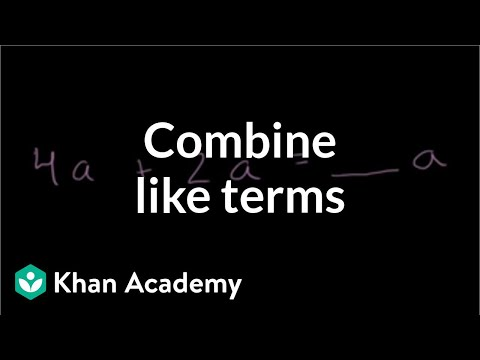COVID-19 presents an unprecedented challenge for teachers, students, and parents. Explore resources from IBM and partners here.
Video

# Evaluating expressions with variables: temperature (Full video)

Khan Academy

## Supporting grades: 6

Description: In this example we have a formula for converting Celsius temperature to Fahrenheit. Let's substitute the variable with a value (Celsius temp) to get the degrees in Fahrenheit. Express 25 degrees Celsius as a temperature in degrees Fahrenheit using the formula Fahrenheit, or F, is equal to 9/5 times the Celsius degrees plus 32. So we could put that in for C here, and we'll get the temperature in Fahrenheit degrees. Or it's equal to 45 plus 32 is 77, so this is 77 degrees Fahrenheit.

You must log inorsign upif you want to:*

*Teacher Advisor is 100% free.

### Other videos you might be interested in### Combining like terms (Full video)

#### Khan Academy